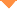首页 > 论文 > 光学学报 > 40卷 > 18期(pp：1806006--1)

Orthogonal Frequency Division Multiplexing Vector Millimeter-Wave Generation Based on Two Parallel Phase Modulators

• 摘要• 论文信息• 参考文献• 被引情况• PDF全文Abstract

We propose an implementation scheme for generating orthogonal frequency division multiplexing (OFDM) vector millimeter-wave (mm-wave) based on two parallel phase modulators (PMs). Compared with previous schemes, the proposed scheme only needs two PMs without complex circuits to control the direct current bias of the modulator and precoding technology, which greatly reduces the complexity of the transmitter and the system cost. The OFDM vector signal and radio frequency (RF) signal are generated by digital signal processing (DSP) module to ensure the flexible adjustment of RF signal frequency. In the DSP module, one up-converted OFDM vector signal and the unmodulated radio frequency signal are transformed by Hilbert transform to obtain the dual single sideband (SSB) signals, respectively, which drive phase modulators. An optical interleaver is used to filter out optical carriers, and a high-speed photodetector is used to obtain the OFDM vector mm-wave signal through beat frequency. Based on the proposed scheme, we demonstrate the generation of a 50-GHz quadrature phase-shift keying (QPSK)-modulated OFDM vector mm-wave signal by simulation with the MATLAB and the optical communication commercial software. The measured results versus received optical power and phase deviation drift are analyzed. The results show that the bit error rate of the transmission system is less than the hard decision forward error correction threshold of 3.8×10 -3, which shows that the scheme of OFDM vector mm-wave signal generation is feasible.【1】Li F, Li Z B, Zou D D, et al. Optical I/Q modulation utilizing dual-drive MZM for fiber-wireless integration system at Ka-band [J]. Optics Letters. 2019, 44(17): 4235-4238.

【2】Pan X L, Liu X Y, Zhang H X, et al. Independent dual single-sideband vector millimeter-wave signal generation by one single I/Q modulator [J]. Optics Express. 2019, 27(14): 19906-19914.

【3】Wang D F, Tang X F, Xi L X, et al. A filterless scheme of generating frequency 16-tupling millimeter-wave based on only two MZMS [J]. Optics & Laser Technology. 2019, 116: 7-12.

【7】Zhou W, Zhao L, Zhang J, et al. Four sub-channel single sideband generation of vector mm-wave based on an I/Q modulator [J]. IEEE Photonics Journal. 2019, 11(4): 7204409.

【8】Fan S L, Cao C Q, Zeng X D, et al. An RoF system based on polarization multiplexing and carrier suppression to generate frequency eightfold millimeter-wave [J]. Results in Physics. 2019, 12: 1450-1454.

【9】Li Y Y, Wen A J, Zhang Y, et al. Photonic generation of frequency-doubled microwave waveform based on a PDM-MZM modulator [J]. Optics Communications. 2020, 458: 124756.

【10】Xiao J N, Feng X X, Zhou W, et al. Generation of (3, 1) vector signals based on optical carrier suppression without pre-coding [J]. Optics Letters. 2020, 45(4): 1009-1012.

【11】Li Y Y, Wen A J, Zhang W, et al. A simple and tunable photonic generation of frequency-doubled triangular waveform based on two cascaded modulators [J]. Optics Communications. 2019, 445: 231-235.

【12】Zhao L, Xiong L, Liao M X, et al. QPSK vector millimeter-wave signal generation based on odd times of frequency without precoding [J]. IEEE Photonics Journal. 2018, 10(6): 5502109.

【13】Zhao L, Xiong L, Liao M X, et al. W-band 8QAM vector millimeter-wave signal generation based on tripling of frequency without phase pre-coding [J]. IEEE Access. 2019, 7: 156978-156983.

【14】Wang D F, Xi L X, Tang X F, et al. Photonic filterless scheme to generate V-band OFDM vector mm-wave signal without precoding [J]. Optics Communications. 2020, 466: 125663.

【15】Cavalcante L C P, Rommel S, Dinis R, et al. Performance evaluation of wavelet-coded OFDM on a 4.9 Gb/s W-band radio-over-fiber link [J]. Journal of Lightwave Technology. 2017, 35(14): 2803-2809.

【16】Wang X, Yu J J, Cao Z Z, et al. SSBI mitigation at 60 GHz OFDM-ROF system based on optimization of training sequence [J]. Optics Express. 2011, 19(9): 8839-8846.

【17】Tao L, Yu J J, Yang Q, et al. A novel transform domain processing based channel estimation method for OFDM radio-over-fiber systems [J]. Optics Express. 2013, 21(6): 7478-7487.

【18】Xiao J N, Zhang Z R, Li X Y, et al. OFDM vector signal generation based on optical carrier suppression [J]. IEEE Photonics Technology Letters. 2015, 27(23): 2449-2452.

【19】Xiao J N, Li X Y, Xu Y M, et al. W-band OFDM photonic vector signal generation employing a single Mach-Zehnder modulator and precoding [J]. Optics Express. 2015, 23(18): 24029-24034.

【20】Xu Y M, Li X Y, Yu J J, et al. Simple and reconfigured single-sideband OFDM RoF system [J]. Optics Express. 2016, 24(20): 22830-22835.

Wang Dongfei,Tang Xianfeng,Xi Lixia,Zhang Xiaoguang. Orthogonal Frequency Division Multiplexing Vector Millimeter-Wave Generation Based on Two Parallel Phase Modulators[J]. Acta Optica Sinica, 2020, 40(18): 1806006